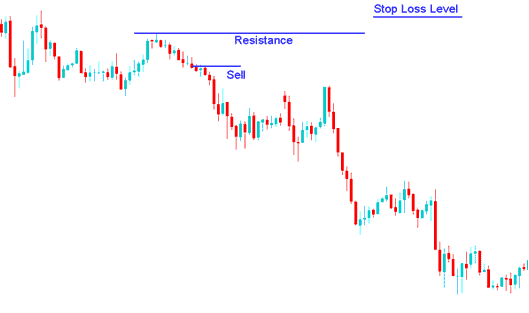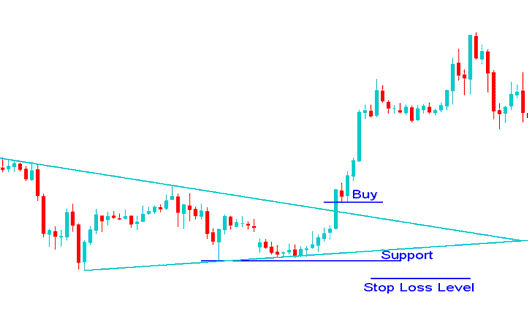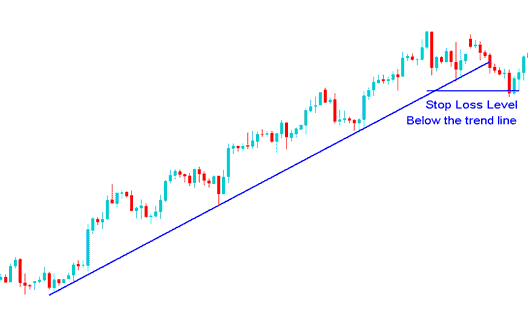# Methods of Setting Stop Loss Orders in Gold Trading

Traders using a Gold trading system must have mathematical calculations that reveal where their stop loss order must be placed.

A Gold trader can also place a stop loss order according to the technical indicators used to set these stop loss orders. Certain technical indicators use mathematical calculations to calculate where the stop loss orders should be set so as to provide an optimal exit point when trading Gold. These chart indicators can be used as the basis for setting these stop loss orders.

Other online Gold traders also place these stop loss orders according to a predetermined risk: reward ratio. This method of setting these orders is dependent upon certain mathematical calculations based on Gold chart price movements. For example a ratio of 50 pips stop loss can be used by a Gold trader if the trade has the potential to make 100 pips in profit; this is a risk reward ratio of 2:1. Also, a ratio of 30 pips stop loss can be used by a Gold trader if the trade has the potential to make 90 pips in profit; this is a risk reward ratio of 3:1

Other traders just use a predetermined percentage of their total trading account balance.

To set a stop loss it is best to use one of the following methods:

## 1. Percentage of trading account balance

This method is based on the percent of the account balance that the trader is willing to risk on any one single Gold trade. If a Gold trader is willing to risk 2% of their trading account balance - then the trader determines how far he will set the stop loss order level based on the position size that he has bought or sold.

Example:

If a trader has a \$100,000 account and is willing to risk 2%

1 pip = \$5

Then setting at 2 %

2% is \$ 2,000

2000 /5 = 400 pips

Stop loss = 400 pips

1 pip = \$10

Then setting the stop at 2%

2% is \$ 2,000

2000 /10 = 200 pips

Stop loss = 200 pips

1 pip = \$20

Then setting at 2 %

2% is \$ 2,000

2000 /20 = 100 pips

Stop loss = 100 pips

## 2. Setting Stop Loss using Support and Resistance Levels

Another way of setting stop loss orders is to use supports and resistance levels, on the Gold trading charts.

Given that stop loss orders tend to congregate at key points, when one of these support or resistance levels is touched by the price, other orders are set off, like dominos. Stop loss orders tend to accumulate just above or below the resistance or support levels, respectively.

A resistance or a support level should act like a barrier for Gold price movement, this is why they are used to set stop losses, if this barrier is broken then the Gold price movement can go towards the opposite direction of the original Gold price trend, but if this barriers (support and resistance levels) are not broken, then the Gold price will continue moving in the intended direction.## Stop Loss Setting using a Resistance LevelSetting Stop Loss Orders above the Resistance Level

## Stop Loss Setting using a Support LevelSetting Stop Loss Orders below the Support Level

## 3. Setting Stop Losses Using Trend Lines

A trend line can be used to set stop losses where the order is set just below the trend line in an upward trend and above the trend line in a downward trend. As long as the trend line holds then a Gold trader will be able to continue making profits while at the same time setting this stop loss order that will lock his profit once the trend line is broken.Setting the stop loss order below the Gold price trend line

Example of where to set this stop loss order using trend lines in an upward trending Gold market.